Search

Numerical Approximation of Zeroes:
The Midpoint Method
(page 1 of 2)You can always find the exact zeroes of a quadratic equation, because you have a formula: The Quadratic Formula. There are formulas for cubic and quartic equations, but they're so complicated that you'll probably never see them, let alone use them. For polynomial equations of degrees higher than 4, there are no general formulas at all.

When you are asked (in an algebra class) to find the zeroes (the x-intercepts, solutions, or roots) of a polynomial equation, you will almost always be given a polynomial that is actually solvable in some straightforward manner. But most polynomials (in "real life") aren't solvable. How do you find their zeroes? You don't, exactly; instead, you find a numerical approximation to some degree of accuracy. (This "to some degree of accuracy" answer is what you get when you punch buttons on your graphing calculator to find the root of a graphed equation.) How does this work?

The secret to approximating zeroes is to use the "continuity property" of polynomials. This property (or "trait") of polynomials says that, if your polynomial equals, say, 5 at some value of x and equals, say, 10 at some other value of x, then the polynomial takes on every value between 5 and 10 because polynomials are continuous (connected) lines. They don't have gaps or jumps or angles or elbows. They don't do anything hinky or unexpected. They're just nice smooth solid curvy lines.

In particular, if your polynomial is negative (below the x-axis) at one spot and positive (above the x-axis) at another spot, then it has to be zero somewhere in between, because zero falls between the negatives and the positives.

 Look at the polynomial function  y = x5 + x3  3x  2: You can quickly see from the graph that the polynomial is negative (below the axis) at x = 1 and positive (above the axis) at x = 2. For x = 1, y = 3; for x = 2, y = 32. Then the polynomial must be zero (it must cross the axis) somewhere in between x = 1 and x = 2. To find the zero, you would start looking inside this interval.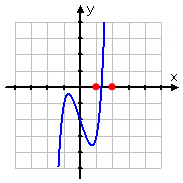In practice, you'll probably be given x-values to use as your starting points, rather than having to find them from a graph. One of the x-values will give a negative value for the polynomial and the other will give a positive value. This is similar to when you use your calculator to find zeroes on a graph, and the calculator asks you to pick left- and right-hand bounds for the zero.

There are two methods for narrowing in on a polynomial's zero: using midpoints, and picking clever values for x. I'll show midpoints first.

• Find the root of the polynomial x5 + x3  3x  2, accurate to three decimal places.
• Right now, I only know that the zero is somewhere between x = 1 and x = 2. To get closer to the zero by using midpoints, I will split the difference, literally: I'll try x = 1.5.

 At x = 1.5, I get y = 4.46875. The actual y-value isn't as important as the sign: since the polynomial is positive here, the graph is above the axis. Since the polynomial is positive at x = 1.5 and x = 2, but is negative at x = 1, I now know that the zero has to be between x = 1 and x = 1.5. (The new interval endpoints, bracketing the zero, are marked in red on the graph.)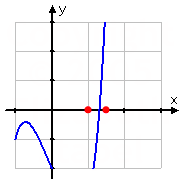So I'll split the difference again. The halfway point between x = 1 and x = 1.5 is x = 1.25. At this point, y = 0.745117, approximately. The important thing to note is that this is a negative y-value. The polynomial is positive at the x = 1.5 endpoint, so the new bounds on my interval are x = 1.25 and x = 1.5.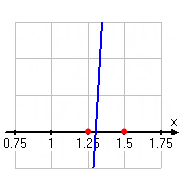Copyright © Elizabeth Stapel 2005-2011 All Rights Reserved   This interval is halved by x = 1.375. (I am taking the average of the two x-values to find my midpoints.) This gives me y = 1.38950, approximately, which is positive. So I'll keep x = 1.25 as the lower endpoint and use x = 1.375 as the new upper boundary.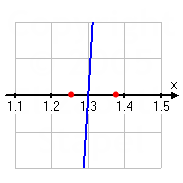The midpoint between x = 1.25 and x = 1.375 is x = 1.3125, which gives y = 0.218389, approximately. Since the polynomial is positive here, I'll keep x = 1.25 as the lower boundary and use x = 1.3125 as the new upper boundary.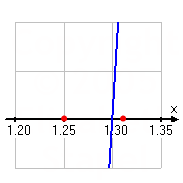The midpoint between x = 1.25 and x = 1.3125 is x = 1.28125, which gives y = 0.287664, approximately. Since the polynomial is negative here, I'll keep x = 1.3125 as the upper boundary and use x = 1.28125 as the new lower boundary.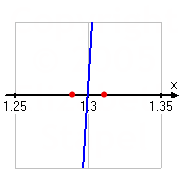The midpoint between x = 1.28125 and x = 1.3125 is x = 1.296875, which gives y = 0.40913, approximately. Since the polynomial is negative here, I'll keep x = 1.3125 as the upper boundary and use x = 1.296875 as the new lower boundary. As you can see, I'm getting a lot closer to where the zero is.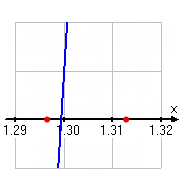The midpoint between x = 1.296875 and x = 1.3125 is x = 1.3046875, which gives y = 0.87143, approximately. Since the polynomial is positive here, I'll keep x = 1.296875 as the lower boundary and use x = 1.3046875 as the new upper boundary.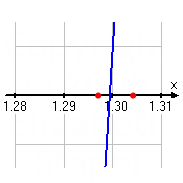The midpoint between x = 1.296875 and x = 1.3046875 is x = 1.30078125, which gives y = 0.0227196, approximately. Since the polynomial is positive here, I'll keep x = 1.296875 as the lower boundary and use x = 1.30078125 as the new upper boundary.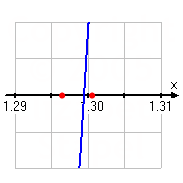You've probably got the idea by now, so I'll just show the rest of my computations:

 Midpoint: 1.298828125y-value: 0.0091952439Lower endpoint: 1.298828125Upper endpoint: 1.30078125 Midpoint: 1.299804688y-value: 0.0067375297Lower endpoint: 1.298828125Upper endpoint: 1.299804688 Midpoint: 1.299316407y-value: 0.0012350122Lower endpoint: 1.299316407Upper endpoint: 1.299804688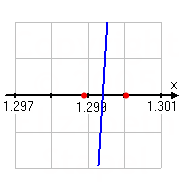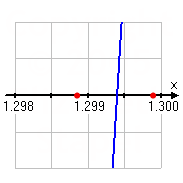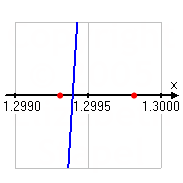The last midpoint becomes the new lower boundary of the interval, and I now have bracketed the polynomial's zero between x = 1.299316407 and x = 1.299804688. Since these two x-values agree in the first three decimal places, I'm done.

The polynomial's zero, to three decimal places of accuracy, is at x = 1.299.